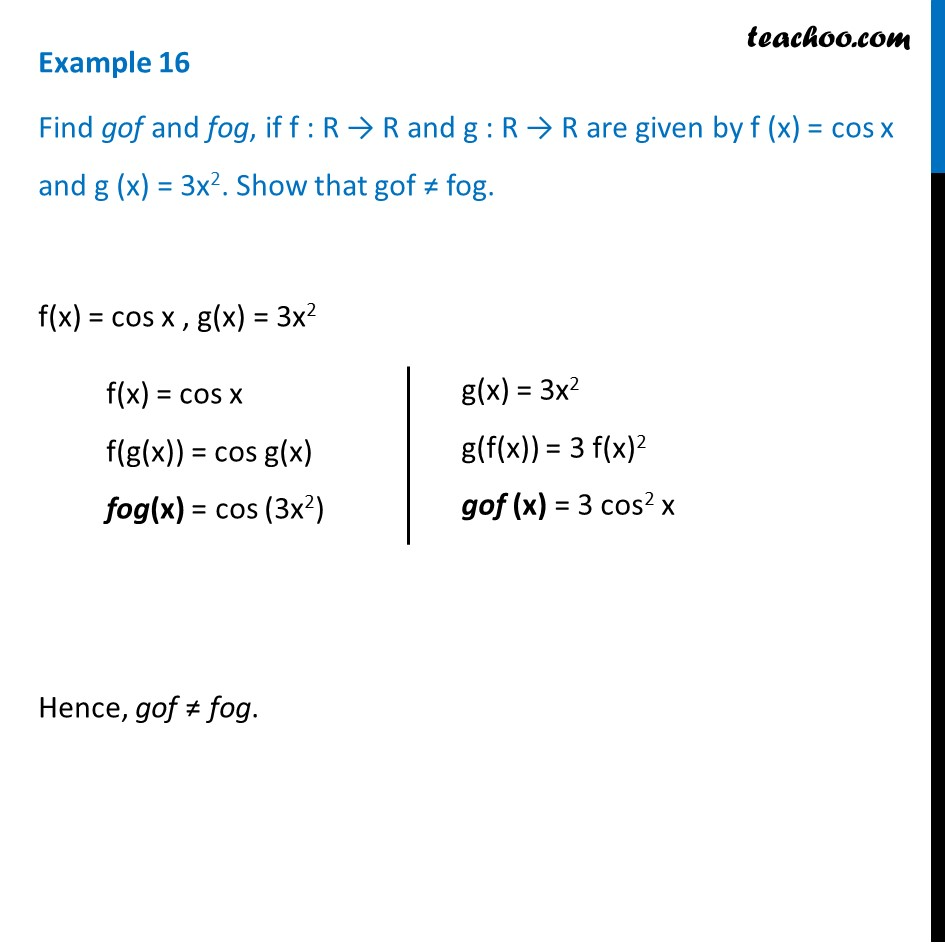Examples

Chapter 1 Class 12 Relation and Functions
Serial order wiseLearn in your speed, with individual attention - Teachoo Maths 1-on-1 Class

### Transcript

Example 16 Find gof and fog, if f : R → R and g : R → R are given by f (x) = cos x and g (x) = 3x2. Show that gof ≠ fog. f(x) = cos x , g(x) = 3x2 Hence, gof ≠ fog. f(x) = cos x f(g(x)) = cos g(x) fog(x) = cos (3x2) g(x) = 3x2 g(f(x)) = 3 f(x)2 gof (x) = 3 cos2 x